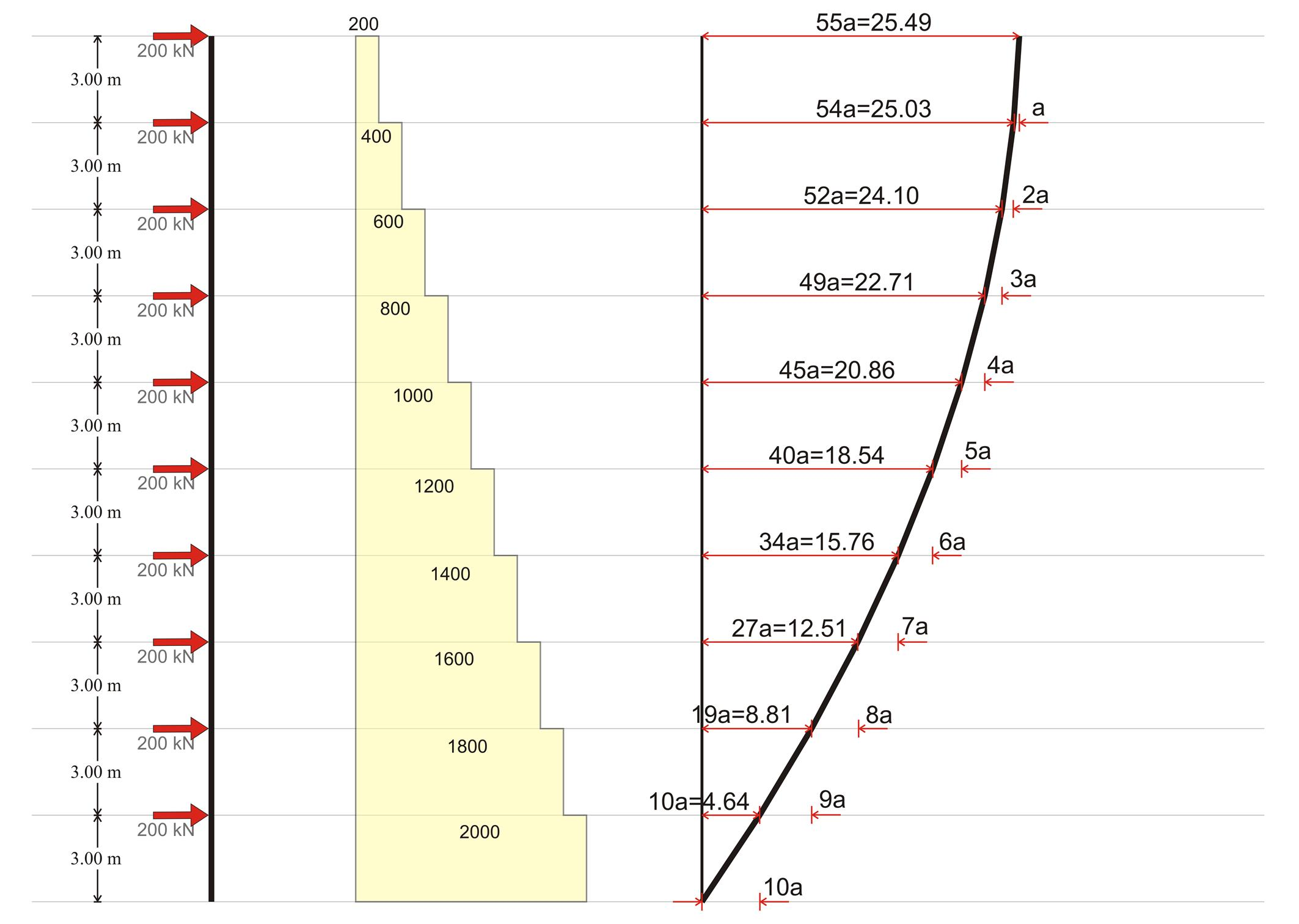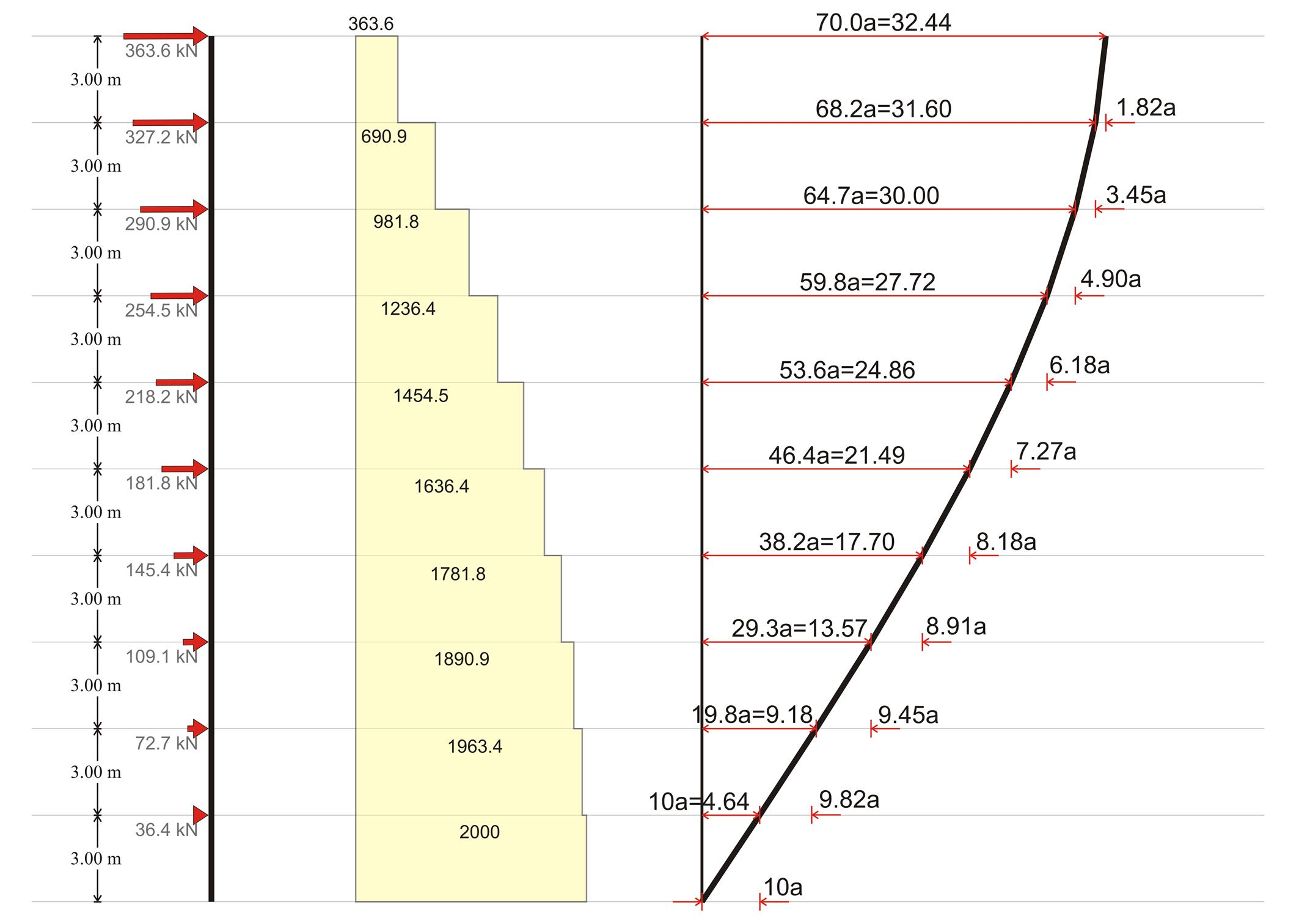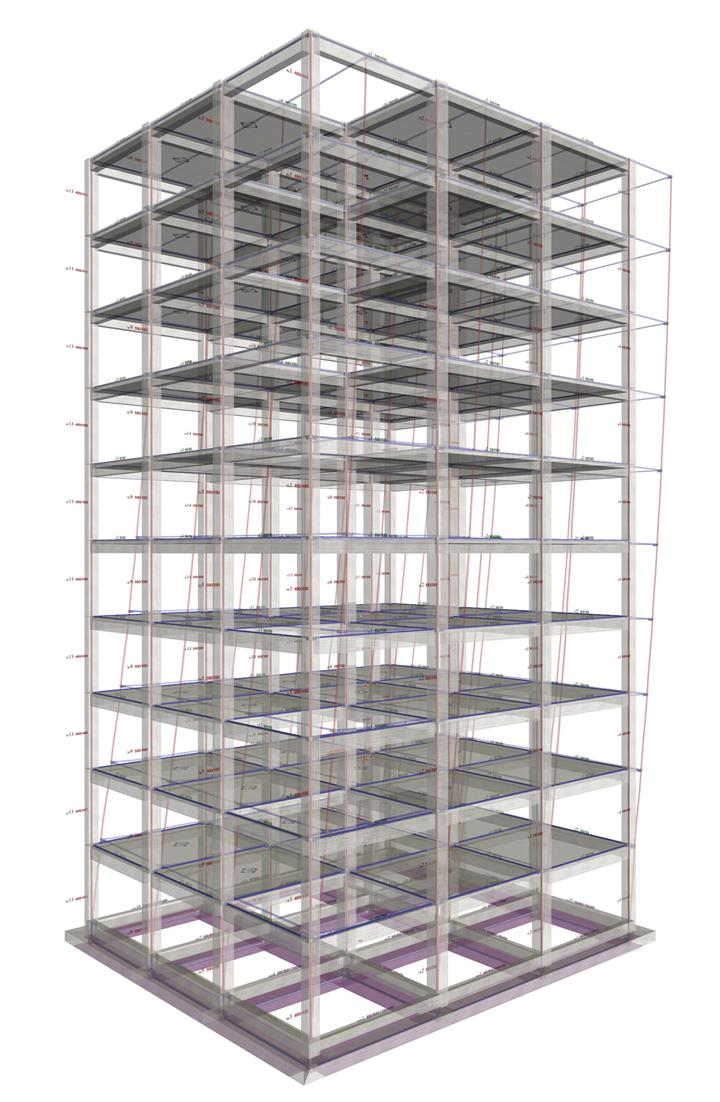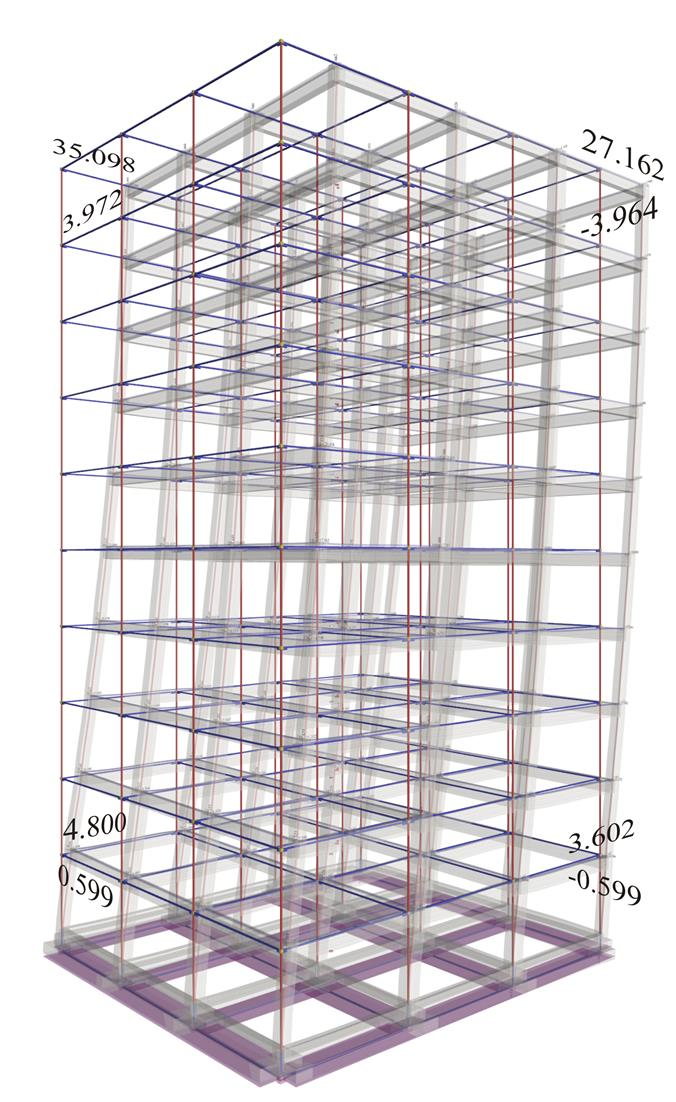« Exercise 1 Exercise 3 »

Exercise 2

In the structure of the previous exercise, assuming that the number of storeys is 10 and the interstorey height is 3.0 m, calculate the displacement the centre of stiffness CT of each floor and the maximum displacement of the higher floor of the building. Consider two cases: (a) orthogonal and (b) triangular distribution of seismic forces.

The displacements deriving from the analysis of the one-storey diaphragm concern the relative displacements between the 1st level floor and the ground.

Assuming that the floor plans are typical and geometrically symmetric the relative stiffness of all floors is the same K=Kxx=Kyy=431.5 MN/m.

The displacement of the CT, called a, of a typical floor under horizontal force H=200 kN, is 200 ·103N /(431.5·106N/m)=0.4635 mm.

The interstorey relative displacement is a function of the storey seismic shear and is equal to a·V/200.

(a) Orthogonal distribution of seismic forces

In the ten-storey building illustrated in the following figure, since all storeys are typical having the same mass, each floor shall be subject to a horizontal seismic force Hi=2000kN/10 Hi=200 (i).

 H [kN] V [kN] δ [mm]Figure 5.4.7.2-1: Base seismic shear = 2000 kN, orthogonal distribution of seismic forces Figure 5.4.7.2-1: Base seismic shear = 2000 kN, orthogonal distribution of seismic forces

At level i: Vi= (11-i)·200 (ii) and the relative displacement with respect to the i-1 level is δzi=(11-i)·a (iii).

The total displacement δi of level i, is the sum of the i terms of the arithmetic progression (Gauss formula) havingas first and last terms δz1=10a and δzi= (11-i) ·a respectively. Therefore

δ i =i · ( δ z1 + δ zi )/2=i · [10a+(11-i) · a]/2 δ i = i · (21-i) · a/2 (iv)

The above figure plots the results of equations (i), (ii), (iii) and (iv).

Conclusions :

• The maximum absolute displacement δ10=55a occurs at the 10th level, and the minimumδ1=10a at the 1st level. On the contrary, the maximum relative displacement δz1=10a occurs at the 1st level, and the minimum δz10=a at the 10 th level.
• The maximum stress is developed at the 1st level due to the maximum relative displacement, while the minimum at the 10th level.
• Due to non-coincident center of mass CM and center of stiffness CT , the diaphragm moments are proportional to the shearforces and the corresponding displacements are proportional to the CTdisplacements. Therefore the maximum displacement occurs at the 10th level in column c13 and is 55/10=5.5 times greater than the one of the ground floor, i.e. δxx,10,13 =5.5·5.60=30.8 mm andδxy,10,13=5.5·0.97=5.3 mm

(b) Triangular distribution of seismic forces (see §6.3.3)

In the following ten-storey building, since all storeys are typical in terms of masses, i.e. Mi=m, the elevation zCM of the centre of mass CM of the building is

zCM = Σ (Mi · z i )/ Σ (Mi)=m · (3.0+6.0+9.0+12.0+15.0+18.0+21.0+24.0+27.0+30.0)/(10 · m)=

=10·(3.0+30.0)/(2·10)=16.5 m .

At the CM the seismic force is HCM=2000kN/10=200 kN and the seismic force at level i isHi=(zi/zCM)·HCM=(i·3.0/16.5) ·200Hi=36.36·i (i)

 H [kN] V [kN] δ [mm]Figure 5.4.7.2-2: Base seismic shear = 2000 kN, triangular distribution of seismic forces Figure 5.4.7.2-2: Base seismic shear = 2000 kN, triangular distribution of seismic forces

At level i:

Vi=(11-i) · (363.64+36.364 · i)/2=(4000+400i-363.64i-36.364i2 )/2Vi=2000+18.182·i-18.182·i2 (ii)

The relative displacement δzi of level i to level i-1 is

δ zi =(Vi/200) · a=(10.0+0.09091 · i - 0.09091 · i2) · a (iii)

The total displacement δi of the ist level, is δi=δ i-1+δzi (iv)

The above figure plots the results of equations (i), (ii), (iii) and (iv).

Conclusions :

• At the 1st level, all types of seismic force distribution, including the triangular and the orthogonal, give the same displacement and stress.
• Given the same seismic shear, the displacement in the last floor δ10=70a according to the triangular distribution is greater than the respective value δ10=55a of the orthogonal one.
• The area of the shear forces diagram and heights represents the total moment of the floors and is larger in the triangular distribution than in the rectangular one.
• The maximum displacement, developed at the 10th level in column c13, is 70/10=7.0 times greater than the one of the ground floor, i.e.δxx,10,13=7.0·5.60=39.2 mm and δxy,10,13 =7.0·0.97=6.8 mm.

The behaviour of the actual structure under orthogonal and triangular seismic force distribution is subsequently considered. In the related software, in <project B_547-1>, the seismic forces are input in the dialog "Seismic Forces" located at "Parameters", "Horizontal Forces". For orthogonal distribution input Hx=200 at all levels, while for triangular distribution input values from 364.0 to 36.4. Always check "Apply seismic forces"=ON in order to use in the analysis the given seismic forces, instead of the default derived from the modal response spectrum analysis,. To perform the analysis press "Solve Building" and finally to review the results press "Analysis Results".Figure 5.4.7.2-3: The actual structure of the
building with the wireframe model
Figure 5.4.7.2-3: The actual structure of the
building with the wireframe modelFigure 5.4.7.2-4: Seismic action with base shear
2000 kN and orthogonal distribution of seismic actions
Figure 5.4.7.2-4: Seismic action with base shear
2000 kN and orthogonal distribution of seismic actions

Due to the bisymmetric geometry, in each diaphragm, the center of stiffness CT is located almost at the centre of the floor and therefore its displacement is almost equal to the average of the displacements of columns c4, c13.

To compare all cases, the displacements are divided by a=0.4635 mm.

The displacements of the center of stiffness CT and of columns c4 and c13 are listed in the following table:

The values of the displacements for orthogonal and triangular distribution of seismic forces

 c4 c 1 3 CT Level Distribution of seismic forces δxx (mm) δxx/a δxy (mm) δxy/a δxx (mm) δxx/a δxy (mm) δxy/a δxx (mm) δxy=0 δxx/a 1 η orthogonal 3.602 7.8 -0.599 -1.29 4.800 10.4 0.599 1.29 4.201 9.06 triangular 3.804 8.2 -0.612 -1.32 5.025 10.8 0.609 1.31 4.415 9.52 1 0η orthogonal 27.162 58.6 -3.964 -8.55 35.098 75.7 3.972 8.57 31.130 67.2 triangular 34.974 75.5 -5.100 -11.0 45.190 97.5 5.116 11.0 40.082 86.5

Conclusions :

• Orthogonal distribution: The displacements of the center of stiffness CT of the actual structure at the 1st level are 9.5a while the idealised one's is 10a. At the 10th level, the displacements are 55a and 67a respectively.
• Triangular distribution: The displacements of the center of stiffness CT of the actual structure at the 1st level are 9a while the simulated one's is 10a. At the 10th level, the displacements are 70a and 86.5a at the 10th level.
• The value k=5, assigned to the modelled structure, proved to be quite successful.

Notes :

• The triangular distribution approximates closer the distribution of seismic forces derived from the modal response spectrum analysis (see §6.4).
• The displacements calculated correspond to the elastic stiffnesses of uncracked cross-sections, i.e. considering full values of EI (value k=5 instead of 12 reflects the actual frame stiffness with respect to the theoretical fixed-ended stiffness).
• According to the Eurocodes [EC8, §4.3.1], structural members should be considered as cracked, thus their stiffnesses should be taken half of the elastic ones. Their displacements will be almost double of the calculated ones. For example the maximum displacement of column c13 will be almost 2·45.19=90.38 mm.
• Besides the additional displacements due to cracking, even greater displacements are developed due to the plastic behaviour of the structural members. The plastic displacements derive from the displacements of the uncracked members multiplied by the behavior factor q which was taken into account in the calculation of the base shear (see §6.3.4). If for instance, the value of q had been assumed q=2.10 then the plastic displacement would be equal to 2.10·90.38=190 mm.
• The determination of the maximum plastic displacement of a building is necessary in cases of an existing or eventual adjacent structure, e.g. as in attached buildings, to provide for an adequate seismic joint [EC8, §4.4.2.7 & §4.3.4], required to protect them from earthquake-induced pounding. In this case a seismic joint at least 190 mm wide should be provided.

« Exercise 1 Exercise 3 »